# SIGHT DISTANCE CONSIDERATIONS FOR HIGHWAYSReading time: 1 minute

## What is Sight Distance in Highway Engineering?

Sight Distance is a length of road surface which a driver can see with an acceptable level of clarity. It plays an important role in geometric highway design because it establishes an acceptable design speed, based on a driver's ability to visually identify and stop for a particular, unforeseen roadway hazard or pass a slower vehicle without being in conflict with opposing traffic.

### There are 5 types of sight distances:

• Stopping Sight Distance
• Overtaking Sight Distance
• Intermediate Sight Distance
• Sight Distance @ intersections

### Stopping sight distance (NON-passing sight distance)

It is the minimum distance open to the vision of the driver to intended to stop the vehicle to avoid collision with an obstruction(moving or stationary) which is present on the carriage way.

#### Analysis of Stopping sight distance (S.S.D)S.S.D = L1+L2

Where L1 = the distance travelled by vehicle during total reaction time of driver = Lag distance L2 = the distance travelled by the vehicle after the application of brakes = Braking distance

#### Calculation of lag distance

Let us assume vehicle is travelling “V m/s” & “t seconds” is the total reaction time of driver. t = perception time +break reaction time (Note: it is obtained from PIEV theory P=perception, I=intellection, E=emission, V=volition) According to IRC t = 2.5 seconds Therefore Lag distance L1 = V.t = 2.5.V meters

#### Calculation of braking distance

It can be calculated by equating work done in stopping the vehicle to kinetic energy of moving vehicle.

Work done = K.E

F * L2 = 0.5 * m * V2

f * w * L2 = 0.5 * ( w/g) * V2 ( f = Coefficient of longitudinal friction )

Therefore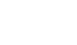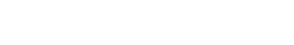(Note: if there is an gradient (n: 100) in the road L2 will change For upward gradient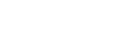For downward gradient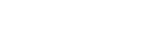“f ” Values As Per IRC
 Design Speed in KMPH Longitudinal Friction (f) 20-30 0.4 40 0.38 50 0.37 60 0.36 65 0.36 80 0.35 100 0.35

### Overtaking Sight Distance (Passing sight distance)

It is the minimum distance open to the vision of the driver intended to overtake a slow moving vehicle safely without causing collision with the opposite vehicle is known as O.S.D.

#### Factors affecting Overtaking Sight Distance:

• Speed of overtaking vehicle ( V m/s)
• Speed of overtaken vehicle ( Vb m/s)
• Speed of opposite vehicle ( V m/s)
• Reaction time of overtaking vehicle driver
• Spacing between the vehicles (S)
• Acceleration of overtaking vehicle (m/s2)

#### Analysis of Overtaking Sight Distance:Overtaking Sight Distance = d1+d2+d3

#### Calculation of d1

d1 = it is a distance travelled by the overtaking vehicle during reaction time (t seconds) of the driver. d1 = Vb.t

#### Calculation of d2

d2 = it is distance travelled by the overtaking vehicle during overtaking time (T seconds) Initial speed = Vb Acceleration = a Time = T seconds Therefore d2 = Vb.T + 0.5*a*T2 From the above fig: d2= b+2s So, b+2s = Vb.T + 0.5*a*T2 Vb.T + 2s = Vb.T + 0.5*a*T2 ( b = speed of over taken vehicle = Vb.T)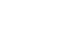According to IRC, “s” is given as S = 0.7Vb+6 Therefore d2 = Vb.T + 2s

#### Calculation of d3

d3 = distance travelled by the opposite vehicle during T seconds d3 = V*T Finally O.S.D = Vb.t + Vb.T + 2s + V.T (NOTE: Vb = V -4.5m/s)

### Intermediate Sight Distance:

It is the sight distance required to provide frequent opportunities of overtaking on highways. Its value is equal to twice S.S.D (stopping sight distance) Therefore, I.S.D = 2 x S.S.D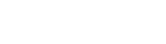H.S.D = S.S.D

### Sight Distance at Interacting Roads:

It is the sight distance required at the junction of two or more roads to avoid collision during crossing of junctions.#### Case1

Enabling the approaching vehicle to change its speed. S.D = V*t

#### Case2

Enabling the approaching vehicle to stop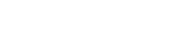#### Case3

Enabling the stopped vehicle to clear of intersection.The greater of above 3 cases will be provide as S.D @ intersections.